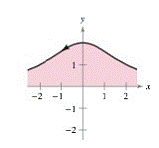Chapter 10.3, Problem 80E

Chapter
Section
Textbook Problem

Area In Exercises 79 and 80, find the area of the region. (Use the result of Exercise 77.) x = 2 cot θ y = 2 sin 2 θ 0 < θ < πTo determine

To Calculate:

The area of the region on the interval 0θπ.

Explanation

Given:

Ellipse of parametric equations:

x=2cotθ

y=2sin2θ and 0θπ

Given graph is:

Formula Used:

If y is a continuous function of x on the interval axb, where x=f(t) and y=g(t), then area of the curve in the interval axb is given by,

abydx=t1t2g(t)f'(t)dt

where f(t1)=a, f(t2)=b and both g and f' are continuous on [t1,t2]

Still sussing out bartleby?

Check out a sample textbook solution.

See a sample solution

The Solution to Your Study Problems

Bartleby provides explanations to thousands of textbook problems written by our experts, many with advanced degrees!

Get Started

Expand each expression in Exercises 122. (y3+2y2+y)(y2+2y1)

Finite Mathematics and Applied Calculus (MindTap Course List)

In Exercises 29-34, rationalize the denominator of each expression. 29. 131

Applied Calculus for the Managerial, Life, and Social Sciences: A Brief Approach

In problems 37-48, compute and simplify so that only positive exponents remain. 39.

Mathematical Applications for the Management, Life, and Social Sciences

If 13f(x)dx=10 and 13g(x)dx=6, then 13(2f(x)3g(x))dx= a) 2 b) 4 c) 18 d) 38

Study Guide for Stewart's Single Variable Calculus: Early Transcendentals, 8th